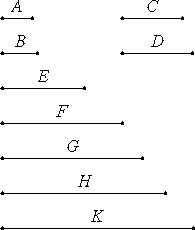# Proposition 2

To find as many numbers as are prescribed in continued proportion, and the least that are in a given ratio.

Let the ratio of A to B be the given ratio in least numbers.

It is required to find numbers in continued proportion, as many as may be prescribed, the least that are in the ratio of A to B.

Let four be prescribed. Multiply A by itself to make C, and by B to make D. Multiply B by itself to make E. Also multiply A by C, D, and E to make F, G, and H, and multiply B by E to make K.VII.17

Now, since A multiplied by itself makes C, and multiplied by B makes D, therefore A is to B as C is to D.

Again, since A multiplied by B makes D, and B multiplied by itself makes E, therefore the numbers A and B multiplied by B make the numbers D and E respectively.

VII.18

Therefore A is to B as D is to E. But A is to B as C is to D, therefore C is to D as D is to E.

VII.17

And, since A multiplied by C and D makes F and G, therefore C is to D as F is to G.

But C is to D as A is to B, therefore A is to B as F is to G.

VII.17

Again, since A multiplied by D and E makes G and H, therefore D is to E as G is to H. But D is to E as A is to B. Therefore A is to B as G is to H.

VII.18

And, since A and B multiplied by E make H and K, therefore A is to B as H is to K. But A is to B as F is to G, and as G is to H. Therefore F is to G as G is to H, and as H is to K.

Therefore C, D, and E, and F, G, H, and K are proportional in the ratio of A to B.

I say next that they are the least numbers that are so.

VII.22

Since A and B are the least of those which have the same ratio with them, and the least of those which have the same ratio are relatively prime, therefore A and B are relatively prime.

VII.27

And the numbers A and B multiplied by themselves respectively make the numbers C and E, and multiplied by the numbers C and E respectively make the numbers F and K, therefore C and E and F and K are relatively prime respectively.

VIII.1

But, if there are as many numbers as we please in continued proportion, and the extremes of them are relatively prime, then they are the least of those which have the same ratio with them. Therefore C, D, and E and F, G, H and K are the least of those which have the same ratio with A and B.

Q.E.D.

# Corollary

From this it is clear that, if three numbers in continued proportion are the least of those which have the same ratio with them, then the extremes are squares, and, if four numbers, cubes.

## Guide

The problem is to construct n numbers in a continued proportion in lowest terms with a given constant ratio. If the ratio is a : b in lowest terms, then the numbers in the continued proportion are

an-1, an-2b, an-3b2, ..., a1bn-2, bn-1

For instance, the five numbers in a continued proportion in lowest terms with a ratio of 2:3 form the sequence 24, 23 . 3, 22 . 32, 2 . 33, and 34, that is, the sequence 16, 24, 36, 54, and 81.

The proof is not difficult. First, adjacent terms do have the correct ratio. Also, since a and b are relatively prime, proposition VII.27 implies that the end terms an-1 and bn-1 are relatively prime. The result then follows from the previous proposition VIII.1.

#### Use of this proposition

This proposition and its corollary are used in several propositions in Book VIII starting with the next and in proposition IX.15 in the next book.